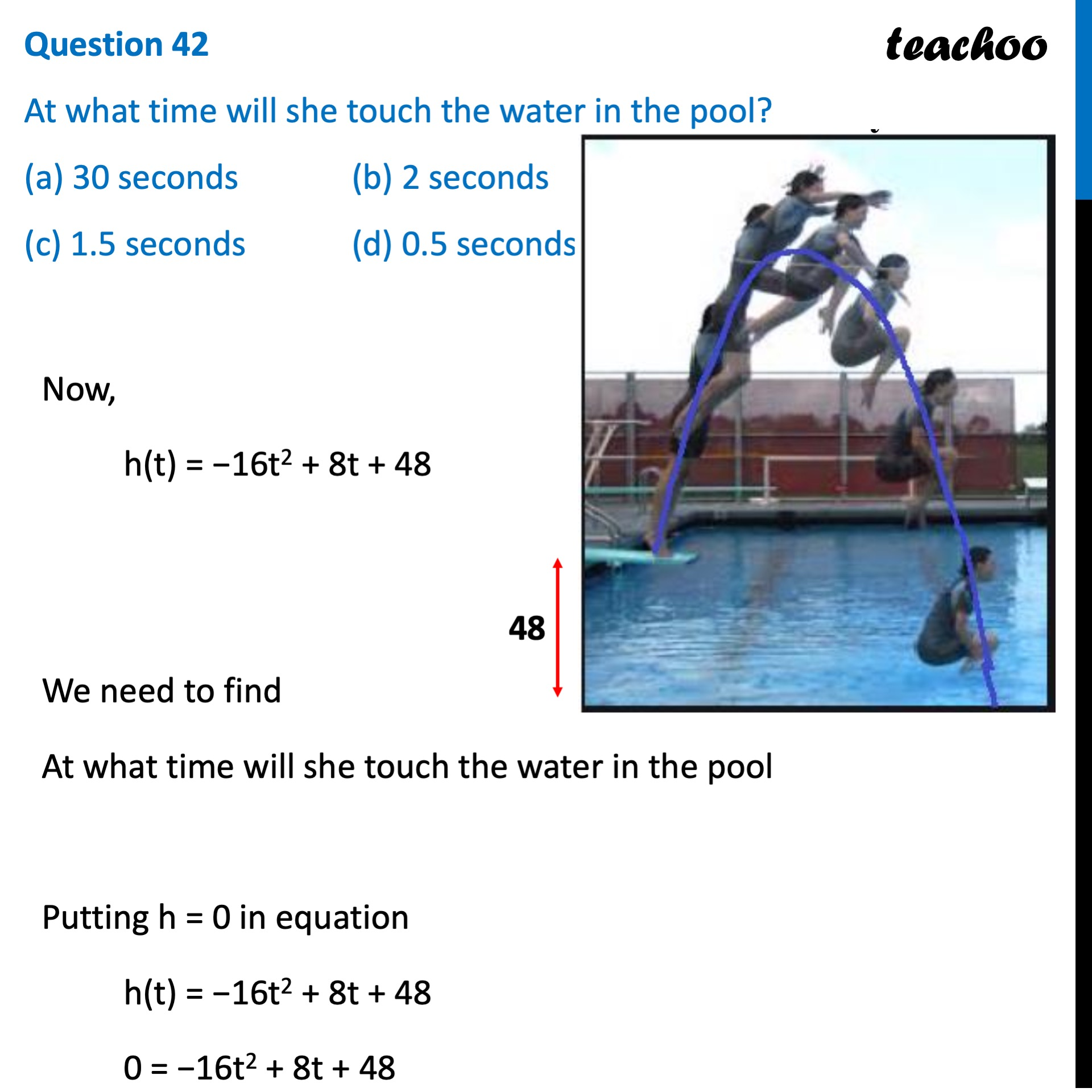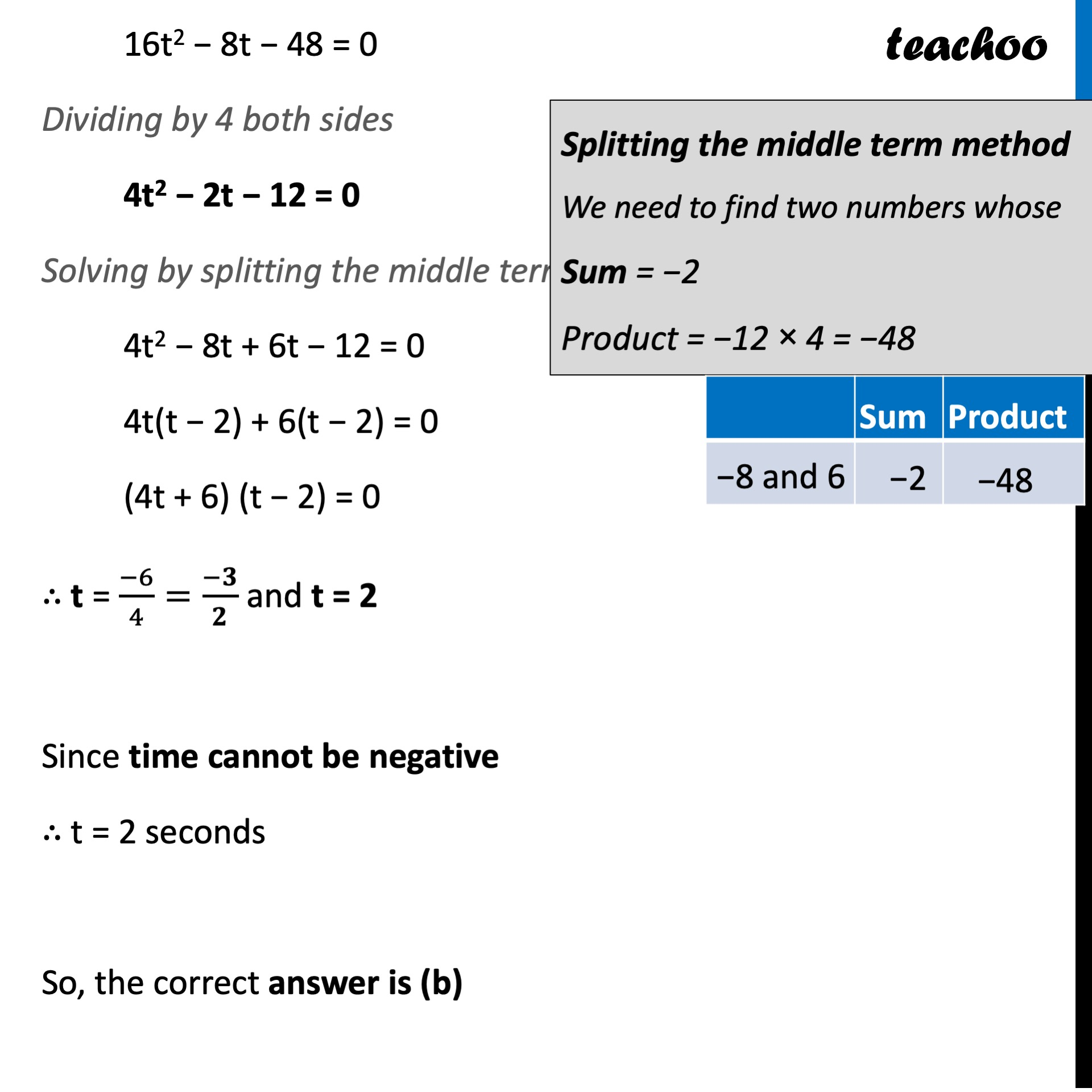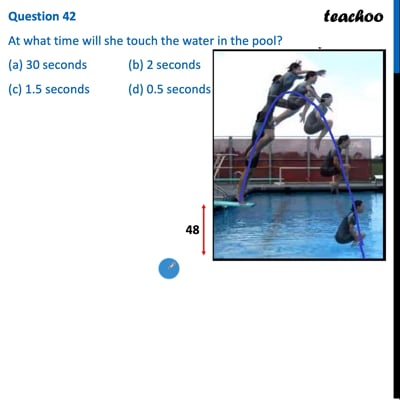CBSE Class 10 Sample Paper for 2022 Boards - Maths Standard [MCQ]

Class 10
Solutions of Sample Papers for Class 10 Boards

## (c) 1.5 seconds   (d) 0.5 secondsThis video is only available for Teachoo black users

Learn in your speed, with individual attention - Teachoo Maths 1-on-1 Class

### Transcript

Question 42 At what time will she touch the water in the pool? (a) 30 seconds (b) 2 seconds (c) 1.5 seconds (d) 0.5 seconds Now, h(t) = −16t2 + 8t + 48 We need to find At what time will she touch the water in the pool Putting h = 0 in equation h(t) = −16t2 + 8t + 48 0 = −16t2 + 8t + 48 16t2 − 8t − 48 = 0 Dividing by 4 both sides 4t2 − 2t − 12 = 0 Solving by splitting the middle term 4t2 − 8t + 6t − 12 = 0 4t(t − 2) + 6(t − 2) = 0 (4t + 6) (t − 2) = 0 ∴ t = (−6)/4=(−𝟑)/𝟐 and t = 2 Since time cannot be negative ∴ t = 2 seconds So, the correct answer is (b) Splitting the middle term method We need to find two numbers whose Sum = −2 Product = −12 × 4 = −48